# Mittag-Leffler summation method

(diff) ← Older revision | Latest revision (diff) | Newer revision → (diff)

A semi-continuous summation method for summing series of numbers and functions, defined by a sequence of functions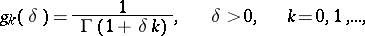whereis the gamma-function. A series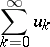is summable by the Mittag-Leffler method to a sumif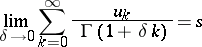and if the series under the limit sign converges. The method was introduced by G. Mittag-Leffler  primarily for the series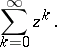A Mittag-Leffler summation method is regular (see Regular summation methods) and is used as a tool for the analytic continuation of functions. Ifis the principal branch of an analytic function, regular at zero and represented by a series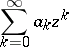for small, then this series is summable by the Mittag-Leffler method toin the whole star of the function(cf. Star of a function element) and, moreover, uniformly in any closed bounded domain contained in the interior of the star.

For summation methods defined by transformations of sequences by semi-continuous matrices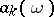of the type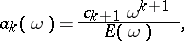whereis an entire function, Mittag-Leffler considered the case when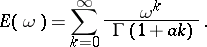A matrixwith such an entire function is called a Mittag-Leffler matrix.

How to Cite This Entry:
Mittag-Leffler summation method. Encyclopedia of Mathematics. URL: http://encyclopediaofmath.org/index.php?title=Mittag-Leffler_summation_method&oldid=12118
This article was adapted from an original article by I.I. Volkov (originator), which appeared in Encyclopedia of Mathematics - ISBN 1402006098. See original article